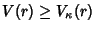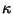## Bishop's Inequality

Letbe the volume of a Ball of radiusin a complete-D Riemannian Manifold with Ricci Curvature. Then, whereis the volume of a Ball in a space having constant Sectional Curvature. In addition, if equality holds for some Ball, then this Ball is Isometric to the Ball of radiusin the space of constant Sectional Curvature.

References

Chavel, I. Riemannian Geometry: A Modern Introduction. New York: Cambridge University Press, 1994.